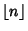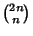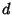## Central Binomial Coefficient

Theth central binomial coefficient is defined as, whereis a Binomial Coefficient andis the Floor Function. The first few values are 1, 2, 3, 6, 10, 20, 35, 70, 126, 252, ... (Sloane's A001405). The central binomial coefficients have Generating FunctionThe central binomial coefficients are Squarefree only for, 2, 3, 4, 5, 7, 8, 11, 17, 19, 23, 71, ... (Sloane's A046098), with no others less than 7320.

The above coefficients are a superset of the alternative central'' binomial coefficientswhich have Generating FunctionThe first few values are 2, 6, 20, 70, 252, 924, 3432, 12870, 48620, 184756, ... (Sloane's A000984).

Erdös and Graham (1980, p. 71) conjectured that the central binomial coefficientis never Squarefree for, and this is sometimes known as the Erdös Squarefree Conjecture. Sárközy's Theorem (Sárközy 1985) provides a partial solution which states that the Binomial Coefficientis never Squarefree for all sufficiently large(Vardi 1991). Granville and Ramare (1996) proved that the only Squarefree values areand 4. Sander (1992) subsequently showed thatare also never Squarefree for sufficiently largeas long asis not too big.''

See also Binomial Coefficient, Central Trinomial Coefficient, Erdös Squarefree Conjecture, Sárközy's Theorem, Quota System

References

Granville, A. and Ramare, O. Explicit Bounds on Exponential Sums and the Scarcity of Squarefree Binomial Coefficients.'' Mathematika 43, 73-107, 1996.

Sander, J. W. On Prime Divisors of Binomial Coefficients.'' Bull. London Math. Soc. 24, 140-142, 1992.

Sárközy, A. On Divisors of Binomial Coefficients. I.'' J. Number Th. 20, 70-80, 1985.

Sloane, N. J. A. Sequences A046098, A000984/M1645, and A001405/M0769, in An On-Line Version of the Encyclopedia of Integer Sequences.'' http://www.research.att.com/~njas/sequences/eisonline.html and Sloane, N. J. A. and Plouffe, S. The Encyclopedia of Integer Sequences. San Diego: Academic Press, 1995.

Vardi, I. Application to Binomial Coefficients,'' Binomial Coefficients,'' A Class of Solutions,'' Computing Binomial Coefficients,'' and Binomials Modulo and Integer.'' §2.2, 4.1, 4.2, 4.3, and 4.4 in Computational Recreations in Mathematica. Redwood City, CA: Addison-Wesley, pp. 25-28 and 63-71, 1991.